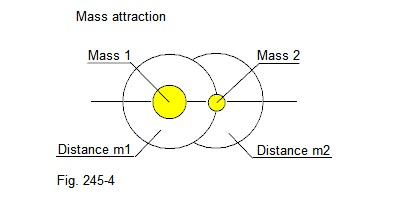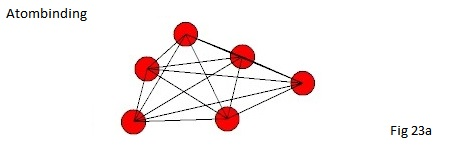Frontpage Search Email . Danish .Welcome to my homepage, developing the classic head physicsBinding molecule. When atomic nuclei are bound together, creating a network of bonds is created which forms a molecule. There are two general types When an atomic nucleus is replaced with another atom core number of a molecular bond, there will be a chemical reaction and there is liberated or absorbed energy, Vol joints are strong. When there is no change, we get a general binding molecule, eg. DNA molecules, and there will only be a minor adjustment of the binding energy, the connectors are weak. It is the atomic nucleus which controls the bond joints between the individual nuclei. There are two parts, one part will try to pull together the atomic nuclei and the second part will try to bump the the cores away. It is the atomic nucleus mass attraction that tries to pull the cores together and it is atomic nuclei radiation that attempts to push away the cores, the common name is the black body radiation pressure and is used in astronomy, Example the sun. Radiation pressure in atomic nuclei is more specific because it is directional specific against each of the atomic nuclei. I am currently working on developing the classic principal physics and nuclear structure. Molecular bonds is about computer simulated models, with some programming work. I therefore have to set priorities. I do not have resources for this development work so far I Would like to give an explanation of how it can be done. There is a huge potential in molecular bonds. You will have knowledge of the exact coordinates set for each atomic nucleus and the strong and weak individual link between the individual cores, and how the whole molecule affects its environments and total control of all chemical molecule compounds. You should be aware that when you work in and around the atomic nucleus it is very large forces acting between the cores, but it can be very small margins that decides the outcome, so be careful with accuracy.I would not recommend it work in joule, but use of mass formula set instead can be downloaded here. Mass is a much simpler way to manage energy levels and makes the programming work much easier. Mass is energy; The type obtained in the context of molecular bonds is of the kinetic energytype, and the energy of atomic nuclei is rest energy. Mass Appeal I show the standard formula for mass attraction The standard formula mx = (V x x c 2 ) / V Where V is the spacer variable and (Vx x c2) is the atom nucleus mass (convected Albert Einsteins formula). mx is the mass attraction from the core of the atom at a given distance. Vx is the atomic nucleus volume, the formulas can be found here The total mass attraction between the two atomic nuclei is the sum of Mass1 + Mass2 mTotal = mM1 + mM2 The unknown factor in this calculation is the distance between the two nuclei and this you need to download in a calculation based on the mole mass. Mass attraction is not thermally dependent and is, therefore,a constant of the distance between two cores You now have the force that pulls together the atomic nuclei, which is the same as trying to bump them off. .....................repulsion The radiation pressure is directionally between the two nucleiIt will take a very long time before it is possible to calculate the radiation pressure from the nuclei precisely, it will also be a clumsy way to do it. Unlike mass attraction, the radiation pressure is temperature dependent, and a calibration is needed to set the parametersize, use the molar mass for it. In addition the molemass volum and mass, the temperature you use be in Kelvin, you must also Correct for the pressure, an ATM. = (9.8) 2 m or form zero point at an atm. programming Once you have completed this, you have a complete set of formulas and you are ready to make your first molecule simulator. The electrons in the molecule have a slight influence on how the bond will proceed and should in the first instance be omitted. Make the program so that in the initial phase there is a great distance between the atomic nuclei and let the program slowly pull them together. The program shall simulate all the links as shown in fig.23a. When all links have the same force each other your molecule is finished. .................The Universe. Classic big bang. Mass formation. Atom structure. Atom binding. Molecular binding. Particle radiation Magnetic fields. Mass attraction. Conclusion Micro physics .Ultima update : 01 april 2017
Copenhagen - Email : info@jwhdk.eu